Miscellaneous

Chapter 5 Class 12 Continuity and Differentiability (Term 1)
Serial order wise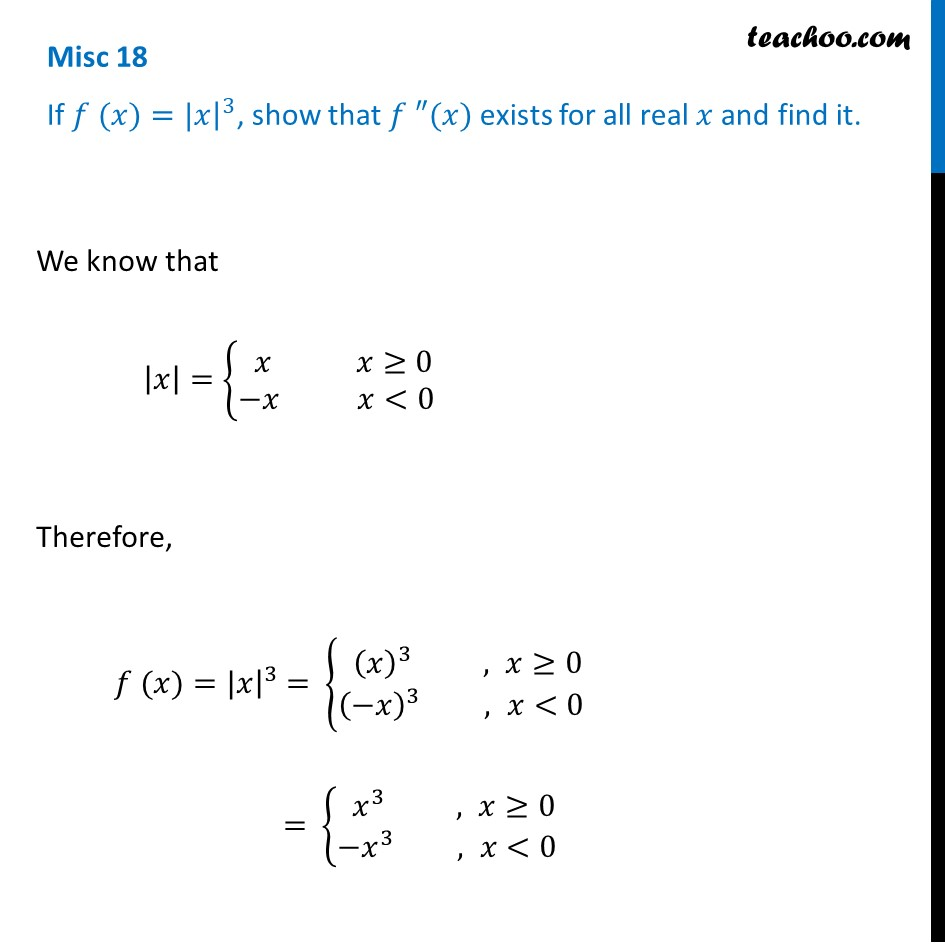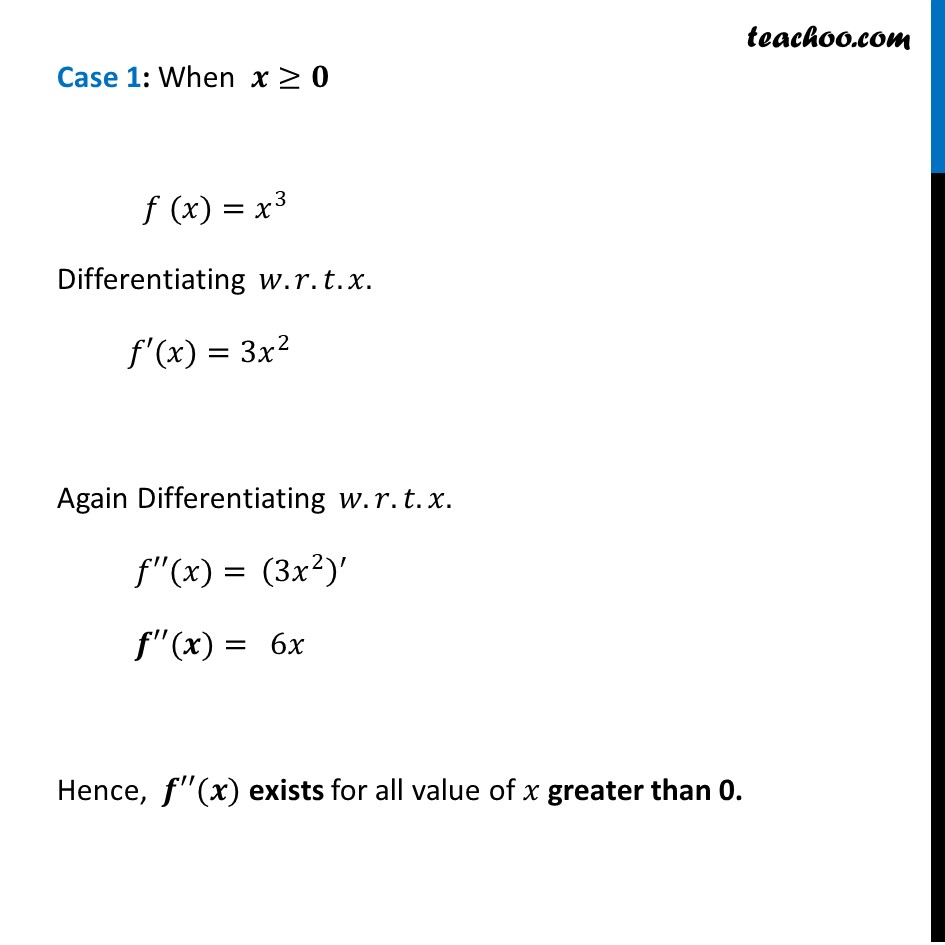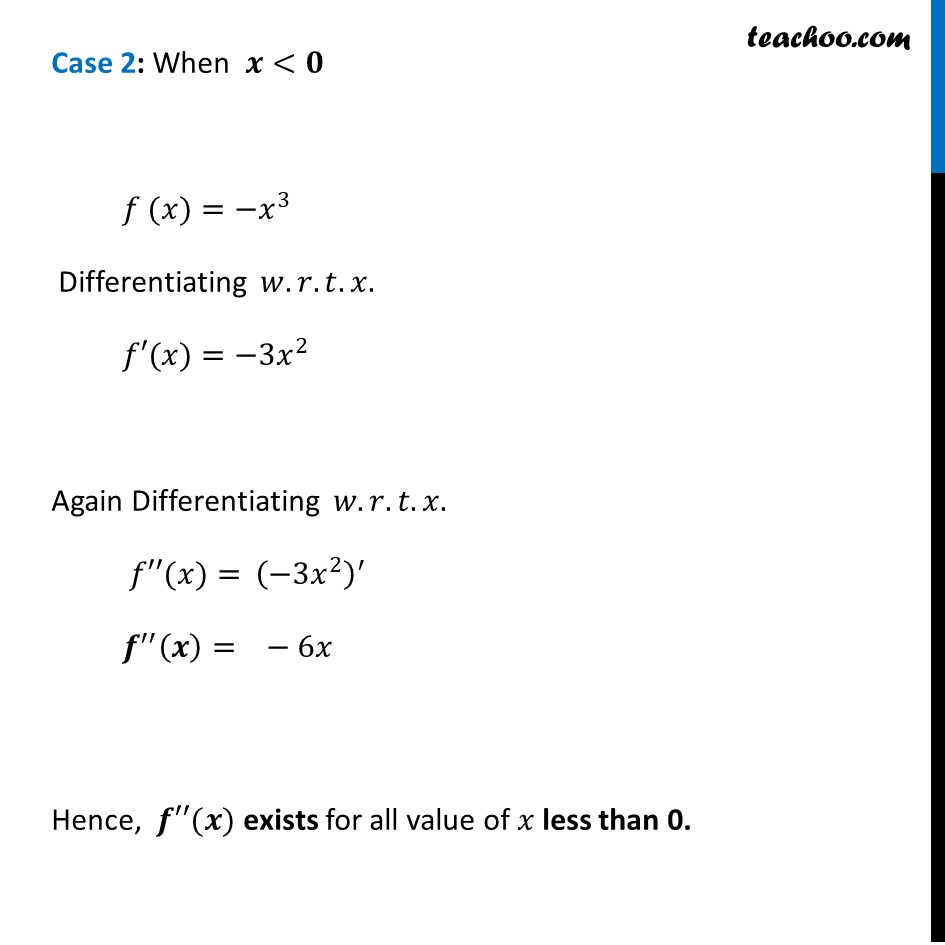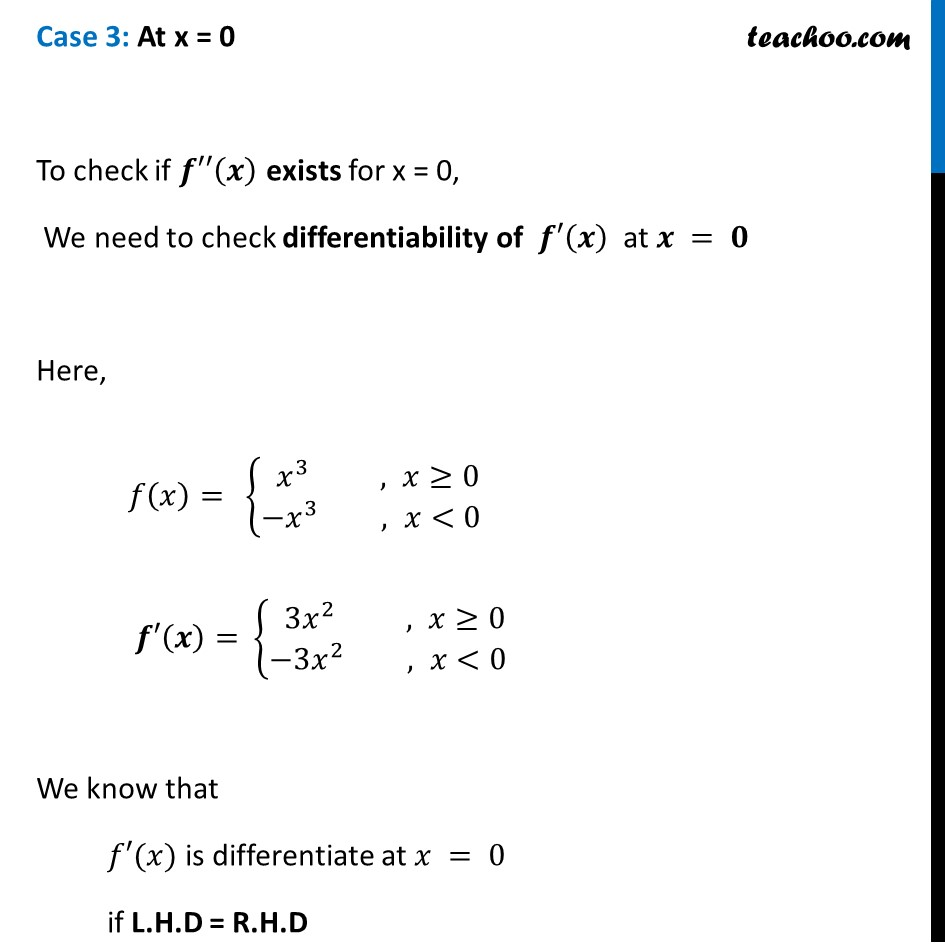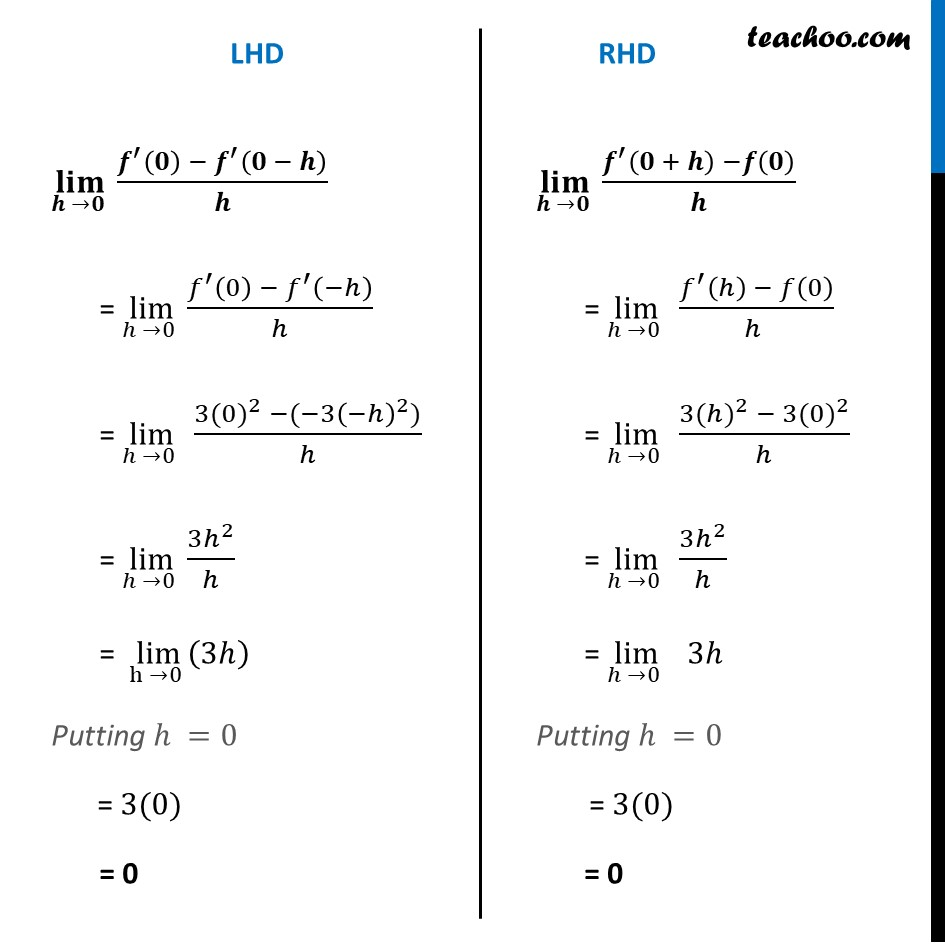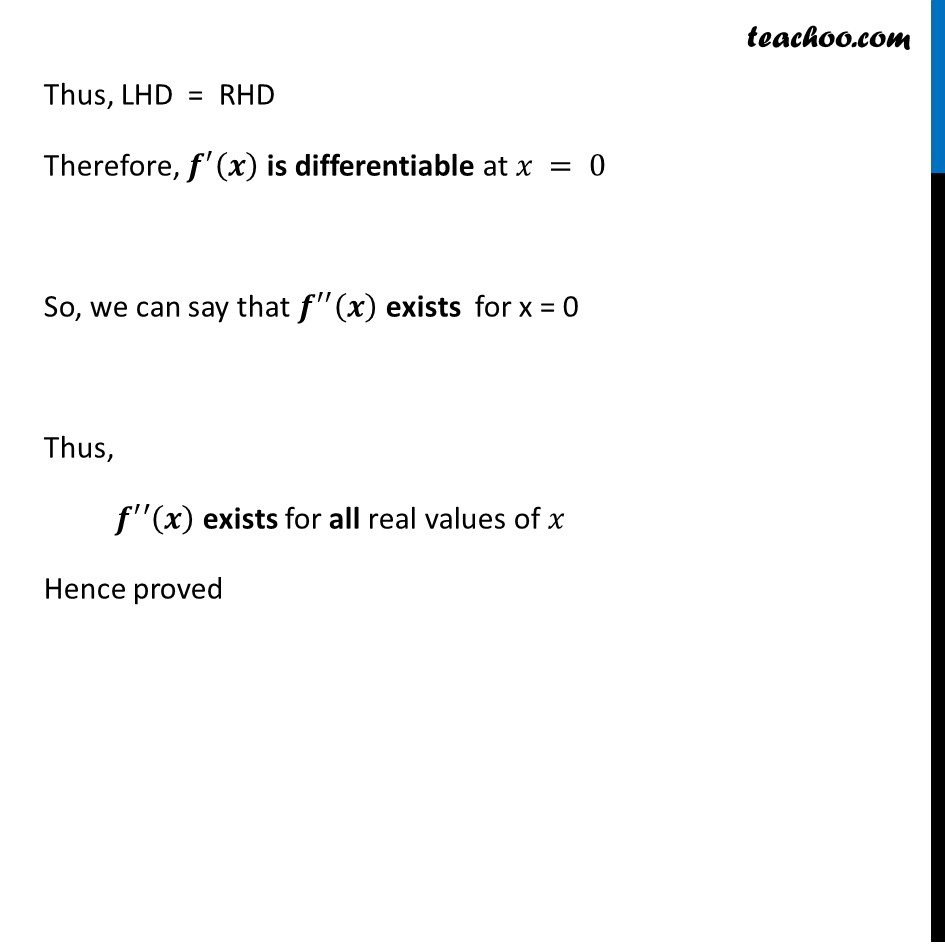### Transcript

Misc 18 If 𝑓 (𝑥)=|𝑥|^3, show that 𝑓 ″(𝑥) exists for all real 𝑥 and find it. We know that |𝑥|={█( 𝑥 𝑥≥0@−𝑥 𝑥<0)┤ Therefore, 𝑓 (𝑥)=|𝑥|^3 = {█( (𝑥)^3 , 𝑥≥0@(−𝑥)^3 , 𝑥<0)┤ = {█( 𝑥^3 , 𝑥≥0@〖−𝑥〗^3 , 𝑥<0)┤ Case 1: When 𝒙≥𝟎 𝑓 (𝑥)=𝑥^3 Differentiating 𝑤.𝑟.𝑡.𝑥. 𝑓′(𝑥)=〖3𝑥〗^2 Again Differentiating 𝑤.𝑟.𝑡.𝑥. 𝑓′′(𝑥)= (3𝑥^2 )^′ 𝒇′′(𝒙)=" " 6𝑥 Hence, 𝒇′′(𝒙) exists for all value of 𝑥 greater than 0. Case 2: When 𝒙<𝟎 𝑓 (𝑥)=〖−𝑥〗^3 Differentiating 𝑤.𝑟.𝑡.𝑥. 𝑓′(𝑥)=〖−3𝑥〗^2 Again Differentiating 𝑤.𝑟.𝑡.𝑥. 𝑓′′(𝑥)= (〖−3𝑥〗^2 )^′ 𝒇^′′ (𝒙)=" "−6𝑥 Hence, 𝒇′′(𝒙) exists for all value of 𝑥 less than 0. Case 3: At x = 0 To check if 𝒇′′(𝒙) exists for x = 0, We need to check differentiability of 𝒇′(𝒙) at 𝒙 = 𝟎 Here, 𝑓(𝑥)= {█( 𝑥^3 , 𝑥≥0@〖−𝑥〗^3 , 𝑥<0)┤ 𝒇′(𝒙)= {█( 〖3𝑥〗^2 , 𝑥≥0@〖−3𝑥〗^2 , 𝑥<0)┤ We know that 𝑓′(𝑥) is differentiate at 𝑥 = 0 if L.H.D = R.H.D(𝐥𝐢𝐦)┬(𝒉 →𝟎 ) (𝒇^′ (𝟎) − 𝒇^′ (𝟎 − 𝒉))/𝒉 = lim┬(ℎ →0 ) (𝑓^′ (0) − 𝑓^′ (−ℎ))/ℎ = lim┬(ℎ →0 ) (〖3(0)〗^2 −(−〖3(−ℎ)〗^2))/ℎ = lim┬(ℎ →0 ) 〖3ℎ〗^2/ℎ = lim┬(h →0 ) (3ℎ) Putting ℎ =0 = 3(0) = 0 (𝐥𝐢𝐦)┬(𝒉 →𝟎 ) (𝒇^′ (𝟎 + 𝒉) −𝒇(𝟎))/𝒉 = lim┬(ℎ →0 ) (〖𝑓𝑎〗^′ (ℎ) − 𝑓(0))/(ℎ ) = lim┬(ℎ →0 ) (〖3(ℎ)〗^2 − 〖3(0)〗^2)/ℎ = lim┬(ℎ →0 ) 〖3ℎ〗^2/ℎ = lim┬(ℎ →0 ) 3ℎ Putting ℎ =0 = 3(0) = 0 Thus, LHD = RHD Therefore, 𝒇^′ (𝒙) is differentiable at 𝑥 = 0 So, we can say that 𝒇^′′ (𝒙) exists for x = 0 a Thus, 𝒇^′′(𝒙) exists for all real values of 𝑥 Hence proved